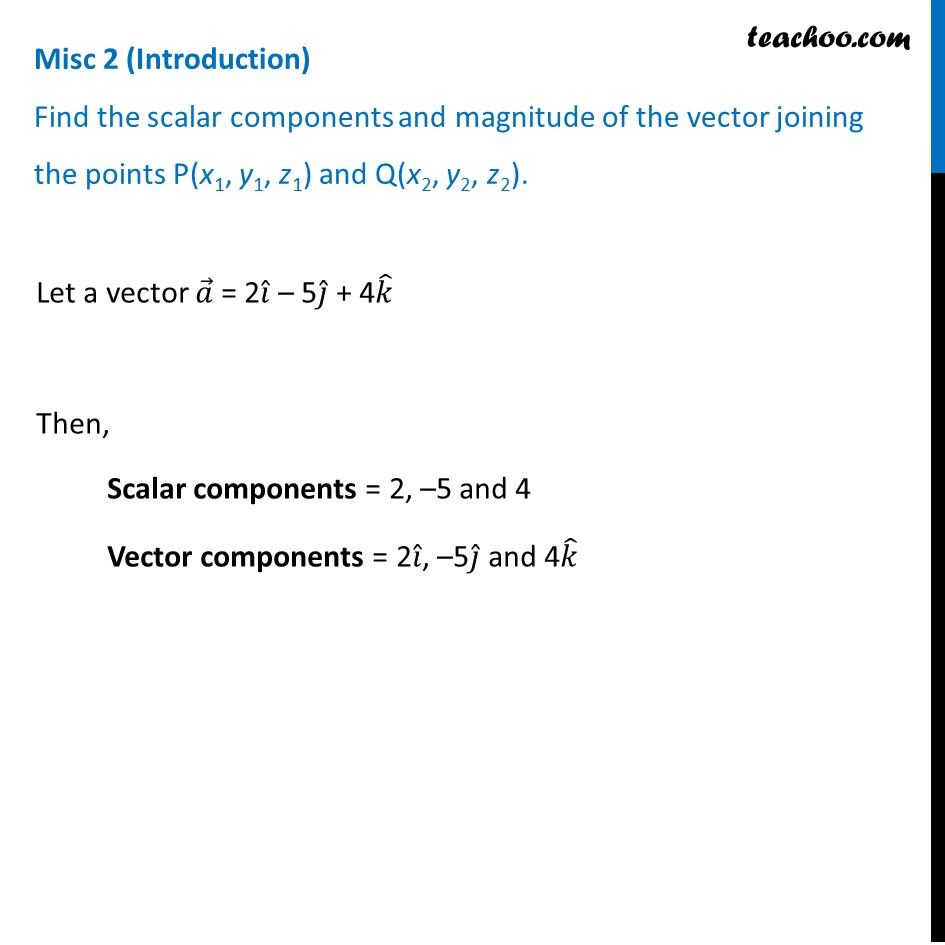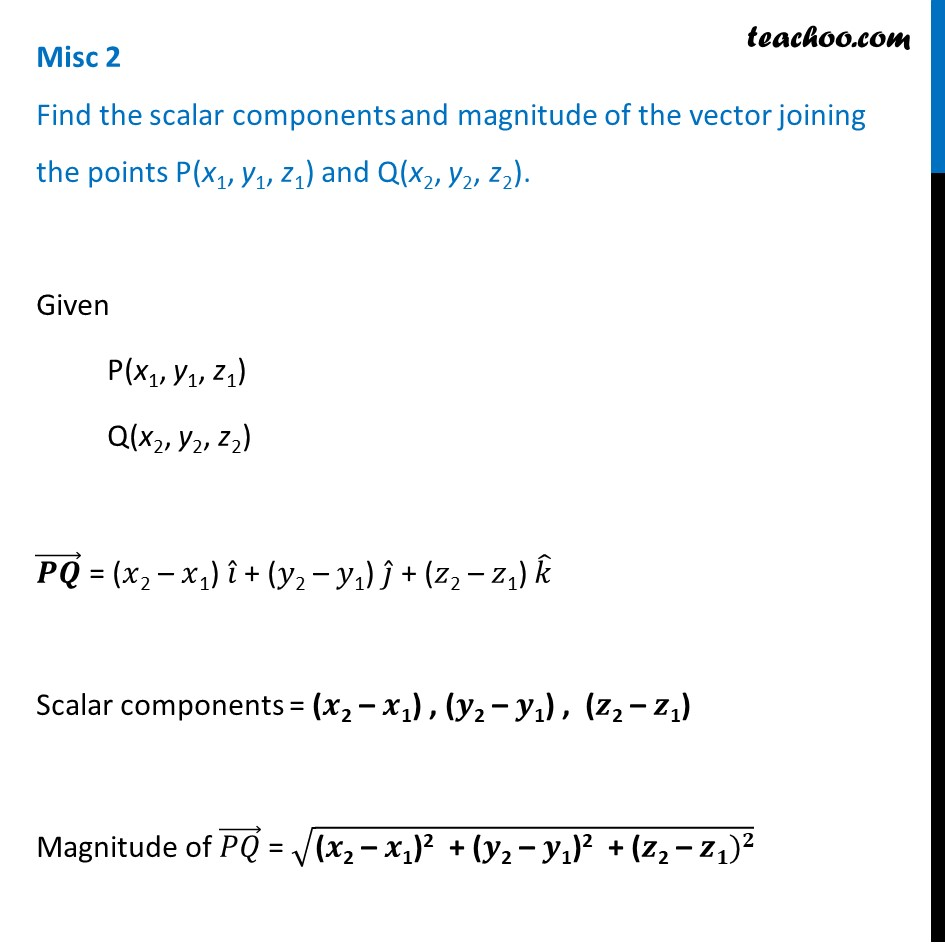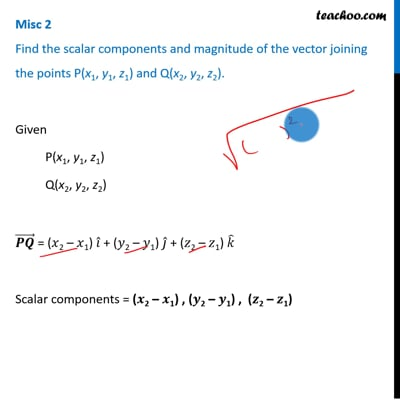Miscellaneous

Chapter 10 Class 12 Vector Algebra
Serial order wiseThis video is only available for Teachoo black usersThis video is only available for Teachoo black users

Introducing your new favourite teacher - Teachoo Black, at only ₹83 per month

### Transcript

Misc 2 (Introduction) Find the scalar components and magnitude of the vector joining the points P(x1, y1, z1) and Q(x2, y2, z2). Let a vector 𝑎 ⃗ = 2𝑖 ̂ – 5𝑗 ̂ + 4𝑘 ̂ Then, Scalar components = 2, –5 and 4 Vector components = 2𝑖 ̂, –5𝑗 ̂ and 4𝑘 ̂ Misc 2 Find the scalar components and magnitude of the vector joining the points P(x1, y1, z1) and Q(x2, y2, z2).Given P(x1, y1, z1) Q(x2, y2, z2) (𝑷𝑸) ⃗ = (𝑥2 – 𝑥1) 𝑖 ̂ + (𝑦2 – 𝑦1) 𝑗 ̂ + (𝑧2 – 𝑧1) 𝑘 ̂ Scalar components = (𝒙2 – 𝒙1) , (𝒚2 – 𝒚1) , (𝒛2 – 𝒛1) Magnitude of (𝑃𝑄) ⃗ = √("(" 𝒙"2 – " 𝒙"1)2 + (" 𝒚"2 – " 𝒚"1)2 + (" 𝒛"2 – " 𝒛𝟏)𝟐)